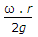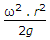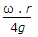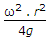# Mechanical Engineering - Hydraulics and Fluid Mechanics

### Exercise :: Hydraulics and Fluid Mechanics - Section 4

6.

The absolute pressure is equal to

 A. gauge pressure + atmospheric pressure B. gauge pressure - atmospheric pressure C. atmospheric pressure - gauge pressure D. gauge pressure - vacuum pressure

Answer: Option A

Explanation:

No answer description available for this question. Let us discuss.

7.

When the pressure intensity at a point is more than the local atmospheric pressure, then the difference of these two pressures is called

 A. gauge pressure B. absolute pressure C. positive gauge pressure D. vacuum pressure

Answer: Option C

Explanation:

No answer description available for this question. Let us discuss.

8.

The hammer blow in pipes occurs when

 A. there is excessive leakage in the pipe B. the pipe bursts under high pressure of fluid C. the flow of fluid through the pipe is suddenly brought to rest by closing of the valve D. the flow of fluid through the pipe is gradually brought to rest by closing of the valve

Answer: Option C

Explanation:

No answer description available for this question. Let us discuss.

9.

When a body is placed over a liquid, it will sink down if

 A. gravitational force is equal to the upthrust of the liquid B. gravitational force is less than the upthrust of the liquid C. gravitational force is more than the upthrust of the liquid D. none of the above

Answer: Option C

Explanation:

No answer description available for this question. Let us discuss.

10.

When a cylindrical vessel of radius (r) containing liquid is revolved about its vertical axis ω rad/s, then depth of parabola which the liquid assumes is

 A.B.C.D.Answer: Option B

Explanation:

No answer description available for this question. Let us discuss.

#### Current Affairs 2021

Interview Questions and Answers